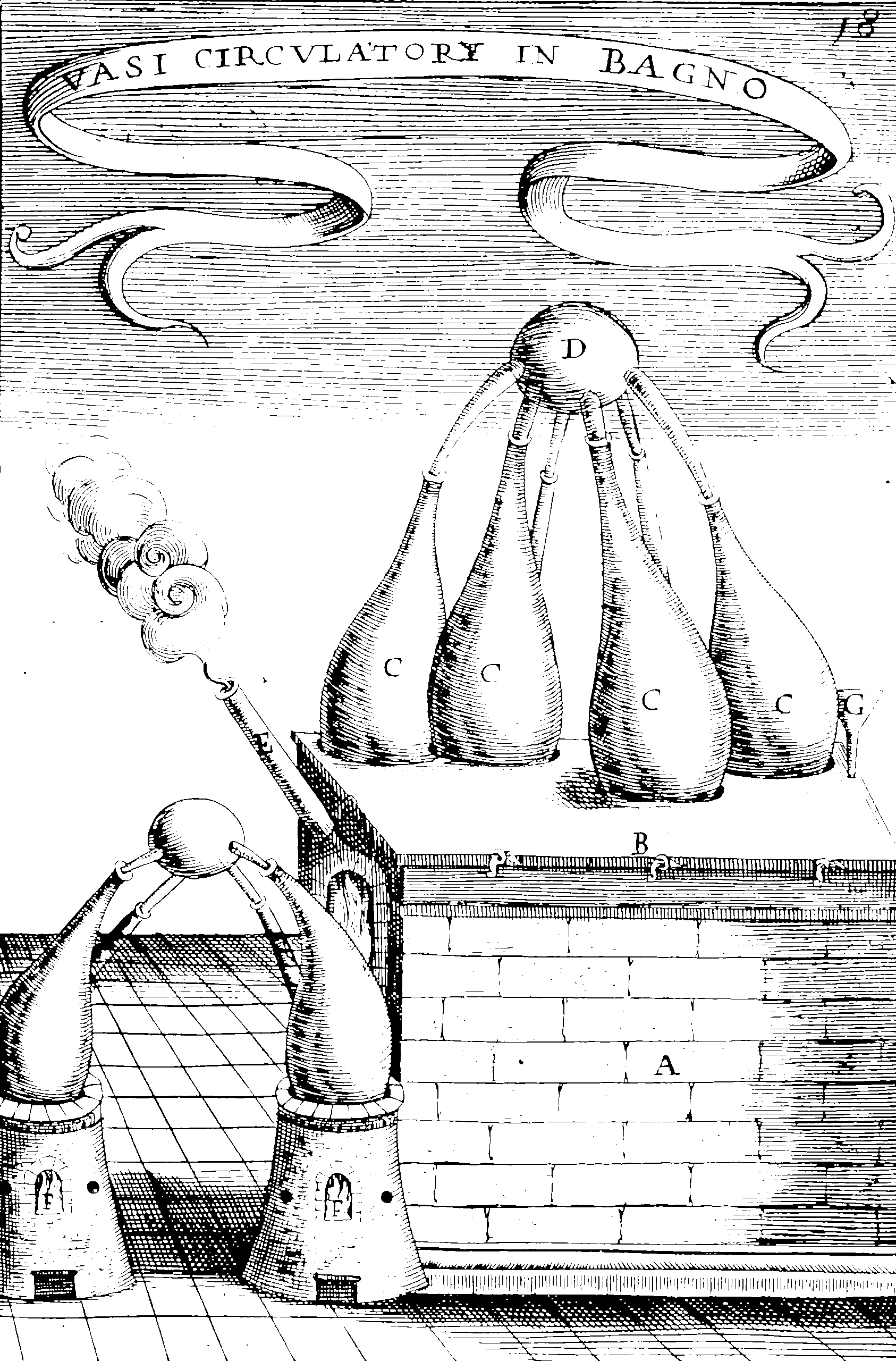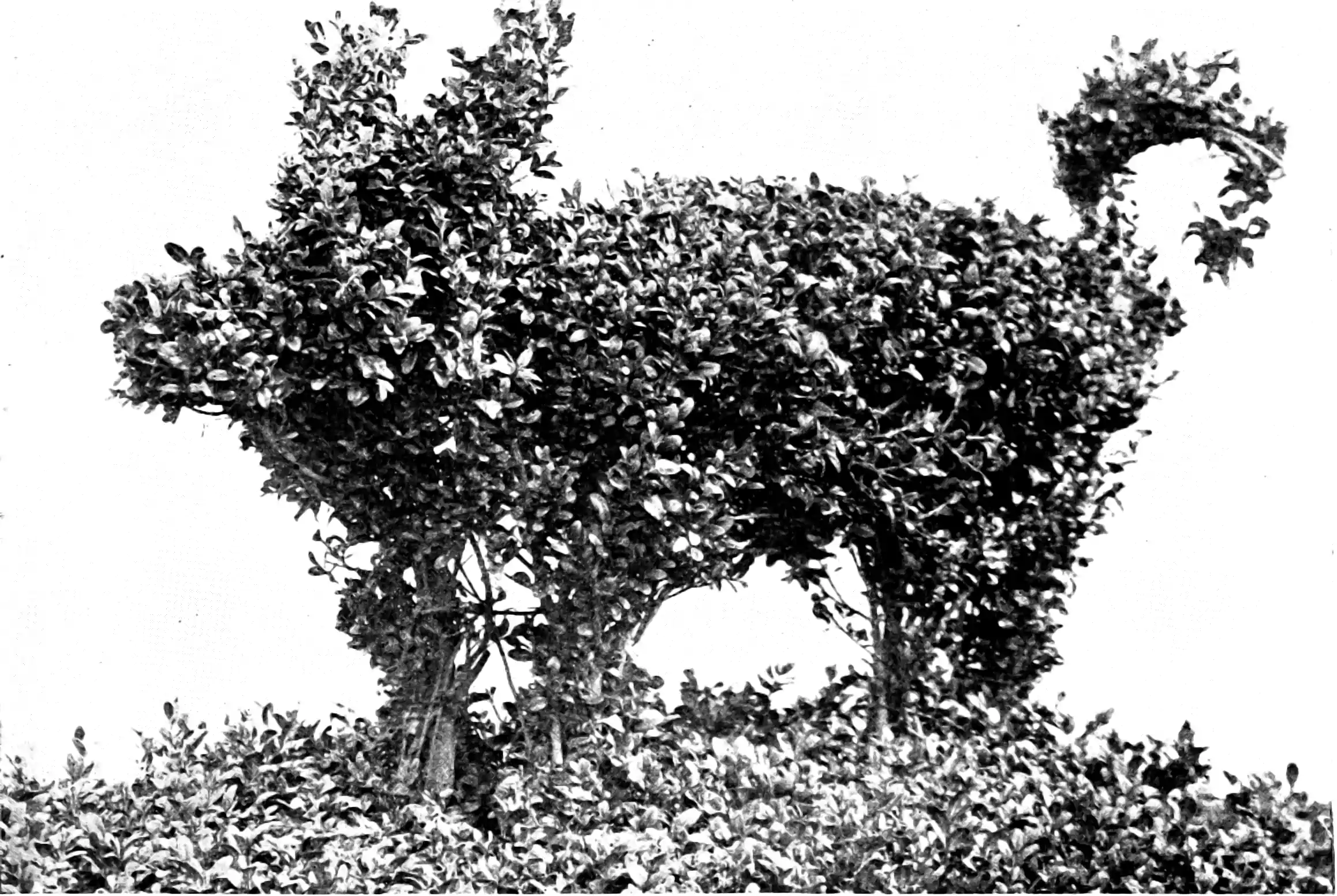# Probabilistic neural nets

## Bayesian and other probabilistic inference in overparameterized ML

Inferring densities and distributions in a massively parameterised deep learning setting.

This is not intrinsically a Bayesian thing to do but in practice much of the demand to do probabilistic nets comes from the demand for Bayesian posterior inference for neural nets. Bayesian inference is, however, not the only way to do uncertainty quantification.

Neural networks are very far from simple exponential families where conjugate distributions might help, and so typically rely upon approximations or luck to approximate our true target of interest.

Closely related: Generative models where we train a process to generate a (possibly stochastic) phenomenon of interest.

## Backgrounders

Radford Neal’s thesis is a foundational Bayesian use of neural networks in the wide NN and MCMC sampling settings. Diederik P. Kingma’s thesis is a blockbuster in the more recent variational tradition.

Alex Graves’ poster of his paper of a simplest prior uncertainty thing for recurrent nets - (diagonal Gaussian weight uncertainty) I found elucidating. (There is a 3rd party quick and dirty implementation.)

One could refer to the 2019 NeurIPS Bayes deep learning workshop site which will have some more modern positioning. There was a tutorial in 2020: by Dustin Tran, Jasper Snoek, Balaji Lakshminarayanan: Practical Uncertainty Estimation & Out-of-Distribution Robustness in Deep Learning.

Generative methods are useful here, e.g. the variational autoencoder and affiliated reparameterization trick. Likelihood free methods seems to be in the air too.

We are free to consider classic neural network inference as sort-of a special case of Bayes inference. Specifically, we interpret the loss function $$\mathcal{L}$$ of a net $$f:\mathbb{R}^n\times\mathbb{R}^d\to\mathbb{R}^k$$ in the likelihood setting \begin{aligned} \mathcal{L}(\theta) &:=-\sum_{i=1}^{m} \log p\left(y_{i} \mid f\left(x_{i} ; \theta\right)\right)-\log p(\theta) \\ &=-\log p(\theta \mid \mathcal{D}). \end{aligned}

Obviously a few things are different here; the parameter vector $$\theta$$ is not interpretable, so what do posterior distributions over it even mean? What are sensible priors? Choosing priors over by-design-uninterpretable parameters such as NN weights is a whole fraught thing that we will mostly ignore for now. Usually it is by default something like $p(\theta)=\mathcal{N}\left(0, \lambda^{-1} I\right)$ for want of a better idea. This ends up being equivalent to the “weight decay” regularisation.

Sweeping those qualms aside, we could do the usual stuff for Bayes inference, like considering the predictive posterior $p(y \mid x, \mathcal{D})=\int p(y \mid f(x ; \theta)) p(\theta \mid \mathcal{D}) d \theta$

Usually this turns out to be intractable to calculate in the very high dimension spaces of an NN, so we encode the net as a simple maximum a posteriori estimate $\theta_{\mathrm{MAP}}:=\operatorname{argmin}_{\theta} \mathcal{L}(\theta).$ In this case we have recovered the classic training of non-Bayes nets. But we have no notion of predictive uncertainty in that setting.

Usually the model will also have many symmetries so we know that it has many optima, which makes approximations that leverage particular modes like a MAP estimate.

Somewhere between the full belt-and-braces Bayes approach and the MAP point estimate there are various approximations to Bayes inference we might try.

🏗 To discuss: so many options for predictive uncertainty, but fewer for inverse uncertainty.

What follows is an non-exhaustive smörgåsbord of options to do probabilistic inference in neural nets.

## MC sampling of weights by low-rank Matheron updates

Needs a shorter names but looks cool .

microsoft/bayesianize: Bayesianize: A Bayesian neural network wrapper in pytorch

## Mixture density networks## Sampling via Monte Carlo

TBD. For now, if the number of parameters is smallish see Hamiltonian Monte Carlo.

## Stochastic Gradient Descent as MC inference

I have a vague memory that this argument is leveraged in Neal (1996)? But see the version in for a highly developed modern take:

Stochastic Gradient Descent with a constant learning rate (constant SGD) simulates a Markov chain with a stationary distribution. With this perspective, we derive several new results.

1. We show that constant SGD can be used as an approximate Bayesian posterior inference algorithm. Specifically, we show how to adjust the tuning parameters of constant SGD to best match the stationary distribution to a posterior, minimizing the Kullback-Leibler divergence between these two distributions.
2. We demonstrate that constant SGD gives rise to a new variational EM algorithm that optimizes hyperparameters in complex probabilistic models.
3. We also propose SGD with momentum for sampling and show how to adjust the damping coefficient accordingly.
4. We analyze MCMC algorithms. For Langevin Dynamics and Stochastic Gradient Fisher Scoring, we quantify the approximation errors due to finite learning rates. Finally,
5. we use the stochastic process perspective to give a short proof of why Polyak averaging is optimal. Based on this idea, we propose a scalable approximate MCMC algorithm, the Averaged Stochastic Gradient Sampler.

A popular recent version of this is the Stochastic Weight Averaging family , which I am interested in. See Andrew G Wilson’s web page for a brief description of the sub methods here, since he seems to have been involved in all of them.

## Laplace approximation

A Laplace approximation locally approximates the posterior using a Gaussian $p(\theta \mid \mathcal{D}) \approx \mathcal{N}\left(\theta_{\mathrm{MAP}}, \Sigma\right).$ Such an approach is classic for neural nets . There are many variants of this technique for different assumptions. Laplace approximations have the attractive feature of providing estimates for forward and inverse problems by leveraging the delta method.

The basic idea is that we hold $$x \in \mathbb{R}^{n}$$ fixed and use the Jacobian matrix $$J(x):=\left.\nabla_{\theta} f(x ; \theta)\right|_{\theta_{\mathrm{MAP}}} \in \mathbb{R}^{d \times k}$$, to the network as $f(x ; \theta) \approx f\left(x ; \theta_{\mathrm{MAP}}\right)+J(x)^{\top}\left(\theta-\theta_{\mathrm{MAP}}\right)$ where the variance is now justifed as a Taylor expansion. Under this approximation, since $$\theta$$ is a posteriori distributed as Gaussian $$\mathcal{N}\left(\theta_{\mathrm{MAP}}, \Sigma\right)$$, it follows that the marginal distribution over the network output $$f(x)$$ is also Gaussian, given by $p(f(x) \mid x, \mathcal{D}) \sim \mathcal{N}\left(f\left(x ; \theta_{\mathrm{MAP}}\right), J(x)^{\top} \Sigma J(x)\right).$ For more on this, see . It is essentially a gratis Laplace approximation in the sense that if I have fit the networks I can already calculate those Jacobians so I am probably 1 line of code away from getting some kind of uncertainty estimate. However, I have no particular guarantees to hope that it is well calibrated, because the simplifications were chosen a priori and might not be appropriate to the combination of model and data that I actually have.

### Learnable Laplace approximations

Agustinus Kristiadi and team have created various methods for low-overhead neural uncertainty quantification via Laplace approximation that have greater flexibility for adaptively choosing the type and manner of approximation. See, e.g. Painless Uncertainty for Deep Learning and their papers .

One interesting variant is that of which generalises to learnable uncertainty to, for example, allow the distribution to reflect uncertainty about outlier datapoints. They define an augmented Learnable Uncertainty Laplace Approximation (LULA) network $$\tilde{f}$$ with more parameters $$\tilde{\theta}=\theta_{\mathrm{MAP}}, \hat{\theta}.$$

Let $$f: \mathbb{R}^{n} \times \mathbb{R}^{d} \rightarrow \mathbb{R}^{k}$$ be an $$L$$-layer neural network with a MAP-trained parameters $$\theta_{\text {MAP }}$$ and let $$\widetilde{f}: \mathbb{R}^{n} \times \mathbb{R}^{\widetilde{d}} \rightarrow \mathbb{R}^{k}$$ along with $$\widetilde{\theta}_{\text {MAP }}$$ be obtained by adding LULA units. Let $$q(\widetilde{\theta}):=\mathcal{N}\left(\tilde{\theta}_{\mathrm{MAP}}, \widetilde{\Sigma}\right)$$ be the Laplace-approximated posterior and $$p\left(y \mid x, \mathcal{D} ; \widetilde{\theta}_{\mathrm{MAP}}\right)$$ be the (approximate) predictive distribution under the LA. Furthermore, let us denote the dataset sampled i.i.d. from the data distribution as $$\mathcal{D}_{\text {in }}$$ and that from some outlier distribution as $$\mathcal{D}_{\text {out }}$$, and let $$H$$ be the entropy functional. We construct the following loss function to induce high uncertainty on outliers while maintaining high confidence over the data (inliers): $\begin{array}{rl} \mathcal{L}_{\text {LULA }}\left(\widetilde{\theta}_{\text {MAP }}\right)&:=\frac{1}{\left|\mathcal{D}_{\text {in }}\right|} \sum_{x_{\text {in }} \in \mathcal{D}_{\text {in }}} H\left[p\left(y \mid x_{\text {in }}, \mathcal{D} ; \widetilde{\theta}_{\text {MAP }}\right)\right] \\ &-\frac{1}{\left|\mathcal{D}_{\text {out }}\right|} \sum_{x_{\text {out }} \in \mathcal{D}_{\text {out }}} H\left[p\left(y \mid x_{\text {out }}, \mathcal{D} ; \widetilde{\theta}_{\text {MAP }}\right)\right] \end{array}$ and minimize it w.r.t. the free parameters $$\widehat{\theta}$$.

I am assuming here that by the entropy functional they mean the entropy of the normal distribution, $H(\mathcal{N}(\mu, \sigma)) = {\frac {1}{2}}\ln \left((2\pi \mathrm {e} )^{k}\det \left({\boldsymbol {\Sigma }}\right)\right)$ but this looks expensive due to that determinant calculation in a (large) $$d\times d$$ matrix. Or possibly they mean some general entropy with respect to some density $$p$$ $H(p)=\mathbb{E}_{p}\left[-\log p( x)\right]$ which I suppose one could estimate as $H(p)=\frac{1}{N}\sum_{i=1}^N \left[-\log p(x_i)\right]$ without taking that normal Laplace approximation at this step, if we could find the density, and assuming the $$x_i$$ were drawn from it.

The result is a slightly weird hybrid fitting procedure that requires two loss functions and which feels a little ad hoc, but maybe it works?

### By stochastic weight averaging.

A Bayesian extension of Stochastic Weight Averaging. ; ; ;

## Via random projections

I do not have a single paper about this, but I have seen random projection pop up as a piece of the puzzle in other methods. TBC.

## In Gaussian process regression

See kernel learning.

See wide NN.

## Via NTK

How does this work? .

## Ensemble methodsDeep learning has its own twists on model averaging and bagging: Neural ensembles. Yarin Gal’s PhD Thesis (Gal 2016) summarizes some implicit approximate approaches (e.g. the Bayesian interpretation of dropout) although dropout as he frames it has become highly controversial these days as a means of inference..

## Practicalities

The computational toolsets for “neural” probabilistic programming and vanilla probabilistic programming are converging. See the tool listing under probabilistic programming.

### No comments yet. Why not leave one?

GitHub-flavored Markdown & a sane subset of HTML is supported.